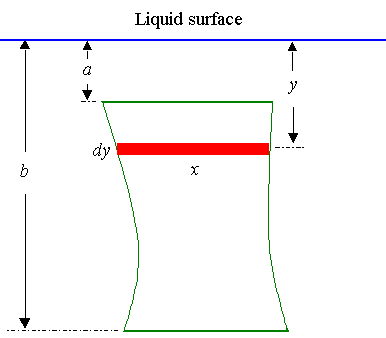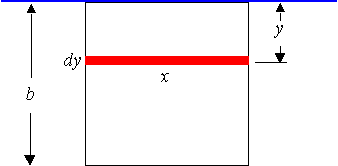Search IntMath
Close

450+ Math Lessons written by Math Professors and Teachers

5 Million+ Students Helped Each Year

1200+ Articles Written by Math Educators and Enthusiasts

Simplifying and Teaching Math for Over 23 Years

# 10. Force Due to Liquid Pressure by Integration

by M. Bourne

The force F on an area A at a depth y in a liquid of density w is given by

F = wyA

The force will increase if the density increases, or if the depth increases or if the area increases.

So if we have an unevenly shaped plate submerged vertically in a liquid, the force on it will increase with depth. Also, if the shape of the plate changes as we go deeper, we have to allow for this.

So we have:Continues below

Now, the total force on the plate is given by

F=wint_a^bxy\ dy

where

x is the length (in m) of the element of area (expressed in terms of y)

y is the depth (in m) of the element of area

w is the density of the liquid (in N m-3)

(for water, this is w = 9800 N m-3)

a is the depth at the top of the area in question (in m)

b is the depth at the bottom of the area in question (in m)

### Example 1

Find the force on one side of a cubical container 6.0 cm on an edge if the container is filled with mercury. The weight density of mercury is 133 kN/m3.

This is the same as having a square plate of sides 6.0 cm submerged in mercury.This is a very basic example where the width of the plate does not change as we move down the plate.

It is always x = 6.

Also, the depth of the top of the plate is 0, so a = 0.

To apply the formula, we have:

x = 0.06\ "m"

y is the variable of integration

w = 133\ "kN m"^-3= 133,000\ "N m"^-3

a = 0

b = 0.06

So we have:

"Force"=w int_a^b xy\ dy

=133000 int_0^0.06 0.06y\ dy

=7980 int_0^0.06\ y\ dy

=7980[(y^2)/(2)]_0^0.06

=14.4\ text[N]

### Example 2

A right triangular plate of base 2.0 m and height 1.0 m is submerged vertically in water, with the top vertex 3.0 m below the surface.

Find the force on one side of the plate.

Before we can proceed, we need to find x in terms of y.

Now when x = 0, y = 3 and when x = 2, y = 4.

So we have:

y=mx+c

=1/2x+3

This gives us x = 2y − 6.

To apply the formula, we have:

x = 2y − 6 = 2(y − 3)

y is the variable of integration

w = 9800\ "N m"^-3

a = 3

b = 4

So we have:

"Force"=wint_a^bxy\ dy

=9800 int_3^4 2y(y-3)\ dy

=19600 int_3^4 (y^2-3y) text[d]y

=19600 [(y^3)/(3)-(3)/(2)y^2]_3^4

=19600[((4^3)/(3)-3/2(4)^2) {:-((3^3)/(3)-3/2(3)^2)]

=19600[-2.667 - (-4.5)]

=35900\ text[N]

## Problem SolverThis tool combines the power of mathematical computation engine that excels at solving mathematical formulas with the power of GPT large language models to parse and generate natural language. This creates math problem solver thats more accurate than ChatGPT, more flexible than a calculator, and faster answers than a human tutor. Learn More.